Download Presentation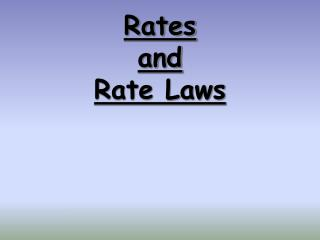Rates and Rate Laws

# Rates and Rate Laws - PowerPoint PPT Presentation

Rates and Rate Laws. Reaction Rate. The change in concentration of a reactant or product per unit of time. Reaction Rates:. 2NO 2 (g)  2NO(g) + O 2 (g). 1. Can measure disappearance of reactants. 2. Can measure appearance of products. 3. Are proportionalI am the owner, or an agent authorized to act on behalf of the owner, of the copyrighted work described.
Download Presentation## Rates and Rate Laws

An Image/Link below is provided (as is) to download presentation

Download Policy: Content on the Website is provided to you AS IS for your information and personal use and may not be sold / licensed / shared on other websites without getting consent from its author.While downloading, if for some reason you are not able to download a presentation, the publisher may have deleted the file from their server.

- - - - - - - - - - - - - - - - - - - - - - - - - - E N D - - - - - - - - - - - - - - - - - - - - - - - - - -
Presentation Transcript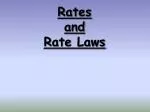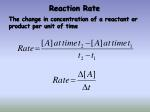Reaction Rate

The change in concentration of a reactant or product per unit of time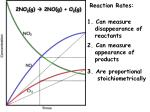Reaction Rates:

2NO2(g)  2NO(g) + O2(g)

1. Can measure

disappearance of

reactants

2. Can measure

appearance of

products

3. Are proportional

stoichiometrically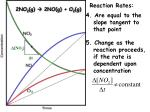Reaction Rates:

2NO2(g)  2NO(g) + O2(g)

4. Are equal to the

slope tangent to

that point

5. Change as the

reaction proceeds,

if the rate is

dependent upon

concentration

[NO2]

t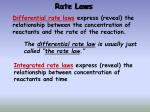Rate Laws

Differential rate lawsexpress (reveal) the relationship between the concentration of reactants and the rate of the reaction.

The differential rate law is usually just called “the rate law.”

Integrated rate lawsexpress (reveal) the relationship between concentration of reactants and time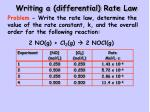Writing a (differential) Rate Law

Problem- Write the rate law, determine the value of the rate constant, k, and the overall order for the following reaction:

2 NO(g) + Cl2(g)  2 NOCl(g)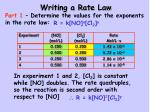Writing a Rate Law

Part 1– Determine the values for the exponents in the rate law:

R = k[NO]x[Cl2]y

In experiment 1 and 2, [Cl2] is constant while [NO] doubles.

The rate quadruples, so the reaction is second order with respect to [NO]

 R = k[NO]2[Cl2]y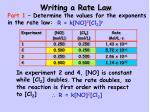Writing a Rate Law

Part 1– Determine the values for the exponents in the rate law:

R = k[NO]2[Cl2]y

In experiment 2 and 4, [NO] is constant while [Cl2] doubles.

The rate doubles, so the reaction is first order with respect to [Cl2]

 R = k[NO]2[Cl2]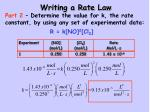Writing a Rate Law

Part 2– Determine the value for k, the rate constant, by using any set of experimental data:

R = k[NO]2[Cl2]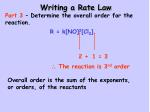Writing a Rate Law

Part 3– Determine the overall order for the reaction.

R = k[NO]2[Cl2]

= 3

2

+

1

 The reaction is 3rd order

Overall order is the sum of the exponents, or orders, of the reactantsDetermining Order withConcentration vs. Time data

(the Integrated Rate Law)

Zero Order:

First Order:

Second Order:Solving an Integrated Rate Law

Problem: Find the integrated rate law and the value for the rate constant, k

A graphing calculator with linear regression analysis greatly simplifies this process!!Time vs. [H2O2]

Regression results:

y = ax + b

a = -2.64 x 10-4

b = 0.841

r2 = 0.8891

r = -0.9429Time vs. ln[H2O2]

Regression results:

y = ax + b

a = -8.35 x 10-4

b = -.005

r2 = 0.99978

r = -0.9999Time vs. 1/[H2O2]

Regression results:

y = ax + b

a = 0.00460

b = -0.847

r2 = 0.8723

r = 0.9340And the winner is… Time vs. ln[H2O2]

1. As a result, the reaction is 1st order

2. The (differential) rate law is:

3. The integrated rate law is:

4. But…what is the rate constant, k ?Finding the Rate Constant, k

Method #1: Calculate the slope from the

Time vs. ln[H2O2] table.

Now remember:

 k = -slope

k = 8.32 x 10-4s-1Finding the Rate Constant, k

Method #2: Obtain k from the linear regresssion analysis.

Regression results:

y = ax + b

a = -8.35 x 10-4

b = -.005

r2 = 0.99978

r = -0.9999

Now remember:

 k = -slope

k = 8.35 x 10-4s-1